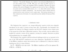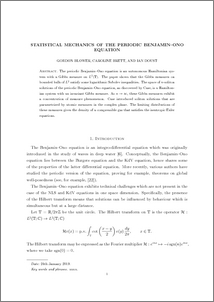# Statistical mechanics of the periodic Benjamin Ono equation

Blower, Gordon and Doust, Ian and Brett, Caroline (2019) Statistical mechanics of the periodic Benjamin Ono equation. Working Paper. UNSPECIFIED. (Unpublished)Preview
PDF (BONO-24Jan2019)
BONO_24Jan2019.pdf

## Abstract

The periodic Benjamin--Ono equation is an autonomous Hamiltonian system with a Gibbs measure on $L^2({\mathbb T})$. The paper shows that the Gibbs measures on bounded balls of $L^2$ satisfy some logarithmic Sobolev inequalities. The space of $n$-soliton solutions of the periodic Benjamin--Ono equation, as discovered by Case, is a Hamiltonian system with an invariant Gibbs measure. As $n\rightarrow\infty$, these Gibbs measures exhibit a concentration of measure phenomenon. Case introduced soliton solutions that are parametrized by atomic measures in the complex plane. The limiting distributions of these measures gives the density of a compressible gas that satisfies the isentropic Euler equat

Item Type:
Monograph (Working Paper)
Uncontrolled Keywords:
/dk/atira/pure/subjectarea/asjc/2600
Subjects:
Departments:
ID Code:
130742
Deposited By:
Deposited On:
25 Jan 2019 11:45
Refereed?:
No
Published?:
Unpublished